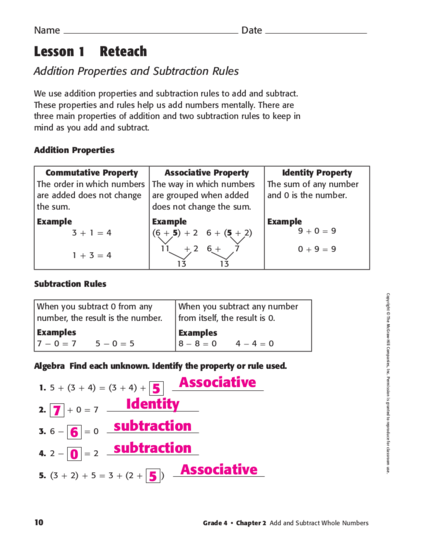# Addition Properties and Subtraction RulesContributed by:Addition Properties and Subtraction Rules. Addition and subtraction are the two primary arithmetic operations where we learn to add and subtract two or more numbers or any mathematical values.
1. Name Date
Lesson 1 Reteach
These properties and rules help us add numbers mentally. There are
three main properties of addition and two subtraction rules to keep in
mind as you add and subtract.
Commutative Property Associative Property Identity Property
The order in which numbers The way in which numbers The sum of any number
are added does not change are grouped when added and 0 is the number.
the sum. does not change the sum.
Example Example Example
3+1=4 (6 + 5) + 2 6 + (5 + 2) 9+0=9
11 +2 6+ 7 0+9=9
1+3=4
13 13
Subtraction Rules
Copyright © The McGraw-Hill Companies, Inc. Permission is granted to reproduce for classroom use.
When you subtract 0 from any When you subtract any number
number, the result is the number. from itself, the result is 0.
Examples Examples
7-0=7 5-0=5 8-8=0 4-4=0
Algebra Find each unknown. Identify the property or rule used.
1. 5 + (3 + 4) = (3 + 4) +5 Associative
2. 7 +0=7 Identity
3. 6 - 6 =0 subtraction
4. 2 - 0 =2 subtraction
5. (3 + 2) + 5 = 3 + (2 + 5) Associative